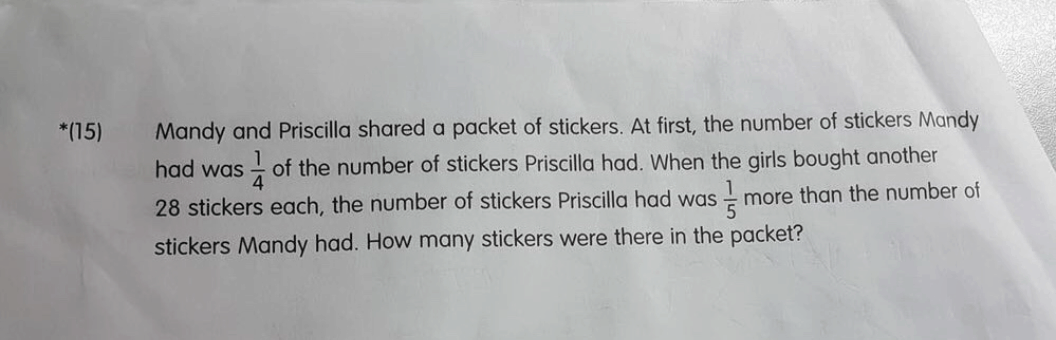# QuestionAt first —
Mandy : 1 unit
Priscilla : 4 units
Total : 5 units

(Note : The number of stickers Priscilla had was 1/5 more than the number of stickers Mandy had is the same as the number of stickers Priscilla had was 6/5 he number of stickers Mandy had)
(1 units + 28) x 6 = 6 units + 168 = (4 units + 28) x 5 = 20 units + 140
1 unit = (168 – 140)/(20 – 6) = 2
5 units = 10

Ans : 10 stickers.

0 Replies 0 Likes

At first —
Mandy : 1 unit
Priscilla : 4 units
Total : 5 units

(Note : The number of stickers Priscilla had was 1/5 more than the number of stickers Mandy had is the same as the number of stickers Priscilla had was 6/5 he number of stickers Mandy had)
(1 units + 28) x 6 = 6 units + 168 = (4 units + 28) x 5 = 20 units + 140
1 unit = (168 – 140)/(20 – 6) = 2
5 units = 10

Ans : 10 stickers.

0 Replies 0 Likes

Identify difference between the two did not change when each gets 28 more stickers.

m : p (before) = 1:4  (Diff is 3 units)

m : p (after) = 5:6    (Diff is 1 part）or multiply by 3 gets 15:18 (diff is 3 units)

increase is 14 units for each girl was due to 28 more stickers.

1 unit is 2 stickers

at first there are 1+4=5 unit in 1 packet, so 10 stickers#

0 Replies 0 Likes